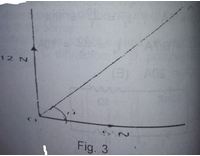# A body of mass 5kg initially at rest is acted upon by two mutually perpendicular forces 12N and 5N as shown in fig.3. If the particle moves in the direction...

Question 1A body of mass 5kg initially at rest is acted upon by two mutually perpendicular forces 12N and 5N as shown in fig.3. If the particle moves in the direction QA, calculate the magnitude of the acceleration